# How to Automatically Increase a Letter by One to Get the Next Letter in Excel?

Sometimes in Excel, you want to number the items by letters instead of numbers, and tying them manually can be a time-consuming process. We can use the automated process to get the result. When we are increasing the value of a number, we can directly use the auto-fill handle, but if we use the auto-fill handle to increase a letter, it will not work. This tutorial will help you understand how we can automatically increase a letter by one to get the next letter in Excel. This tutorial includes two methods one for a single letter and one for a group of letters.

## Automatically Increase Letter by One to Get the Next Letter for a Single Character in Excel

In this case, we enter "A" in the first cell, then the CHAR formula in the cells below, and then use the auto-fill handle. Let us see an effortless process to understand how we can automatically increase letters by one to get the next letter for a single character in Excel.

Step 1

Let us consider a new Excel sheet and enter the first character as shown in the below image.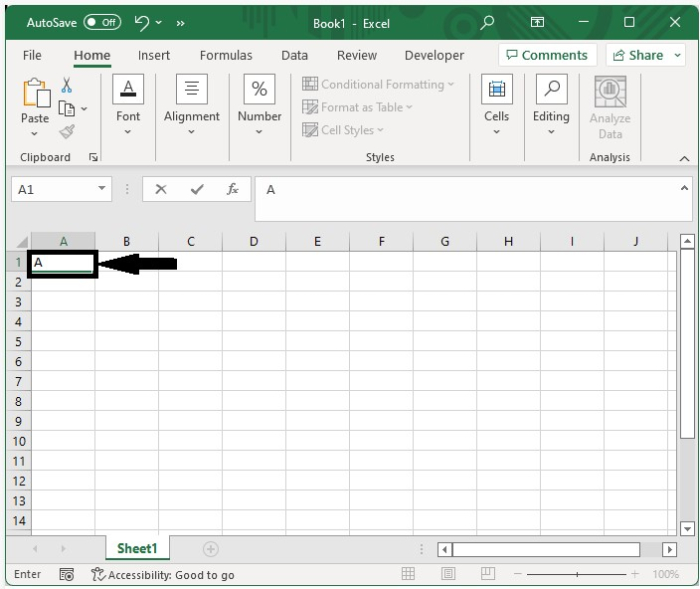To get our first result, click on the cell below and type =CHAR(CODE(A1)+1) into the formula box, then hit enter. In the formula, A1 is the address of already existing data.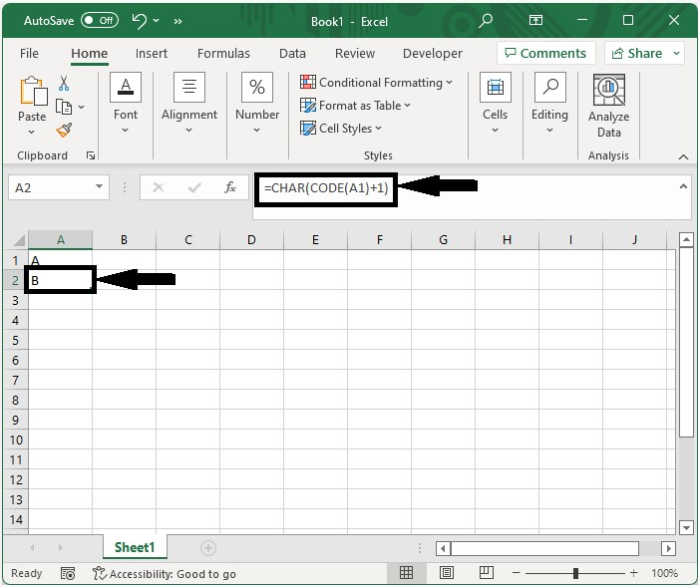Step 2

Now drag down from Cell A2 using the auto-fill handle, and our result will be like the below image.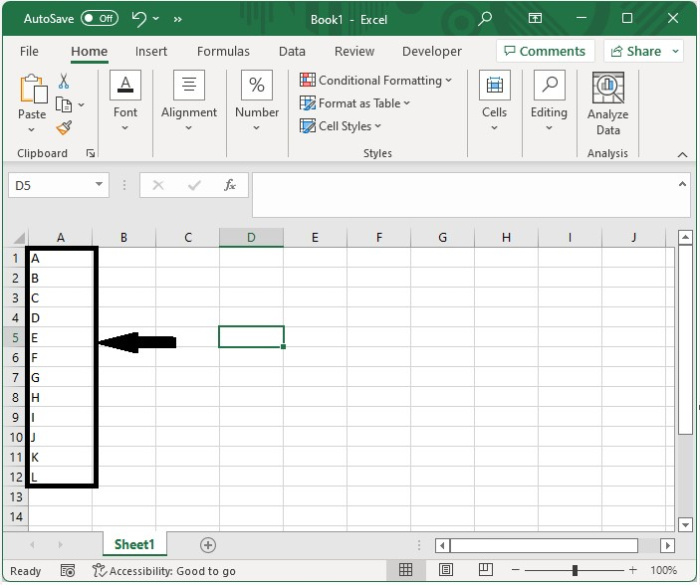## Automatically Increase Letter by One to Get the Next Letter for Multiple Characters

Here we will enter "AA" in the first cell, use the formula, and use the auto-fill handle to complete the task. Let us see an effortless process to know how we can automatically increase letters by one to get the next letter for a combination of characters in Excel.

Step 1

Let us consider a new Excel sheet and enter the first character as shown in the below image.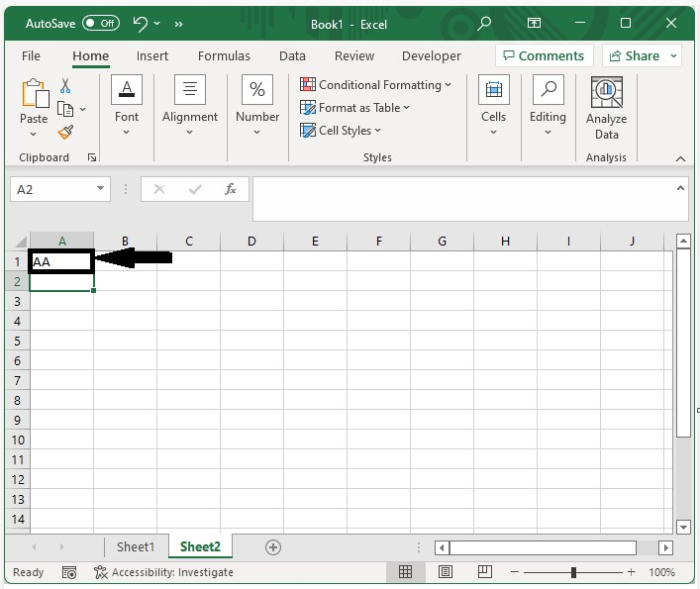Step 2

Now click on the below cell and enter the formula as =IF(RIGHT(\$A1,1)="Z",CHAR(CODE(LEFT(A1,1))+1),LEFT(A1,1)) &CHAR(65+MOD(CODE(RIGHT(A1,1))+1-65,26)) in the formula box and press Enter to get our first result.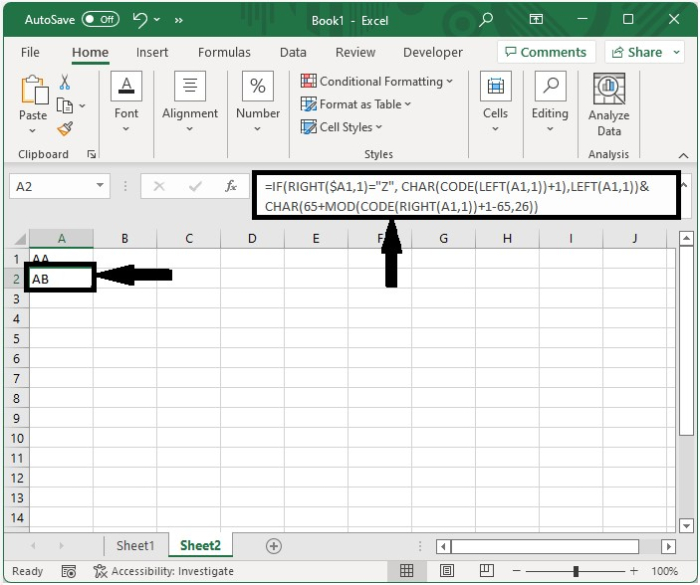Step 3

We can get all the results by dragging down from the first result.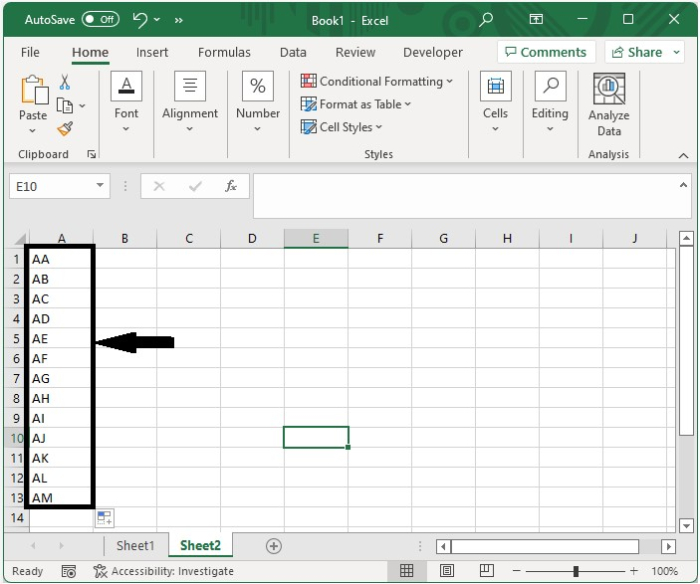## Conclusion

In this tutorial, we used a simple example to show how we can use Excel to highlight a specific set of data by automatically increasing letters by one to get the next letter for single and a combination of characters.

Updated on: 10-Jan-2023

2K+ Views